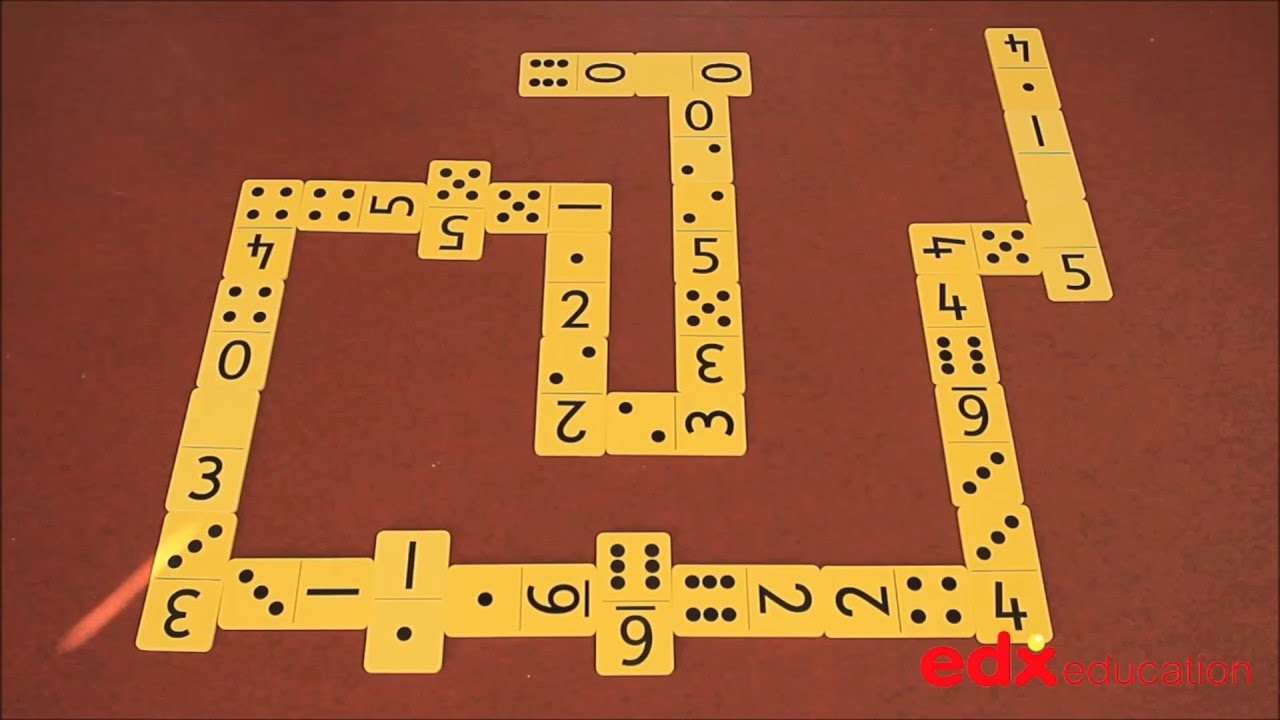# Dominoes

#### • Matching

Dominoes represent all of the different possibilities from throwing two six-faced dice and the extra bonus of a blank to represent zero. The standard game of dominoes involves matching two numbers that are the same. However, dominoes can be the source of much mathematical activity. Students need to be taught the principles of playing dominoes as many practice games are in the form of domino games, for example time dominoes, where the digital and analogue versions of the same time are matched.

### Dominoes VideosIntro – 0:00
Dominoes Sets – 1:30
Domino Dot & Numeral Games –
5:00
Domino Towers –
6:45
Domino Memory –
10:40
Total to 10 – 11:50
Domino Difference – 16:10
Place Value with Dominoes – 17:50
Total to 8 – 22:00
How Dominoes can help fluency –
22:30
Domino Problem Solving –
24:10### Mathematical Language

Double, near double, commutative property, subitising, more than, less than, total.

### Using dominoes

Dominoes may be used to develop early ideas of addition. The spots on one side of the domino may be combined with the spots on the other side of the domino. For example the 6, 3 domino has 9 spots altogether. If the domino is turned around 180 degrees it will show 3, 6 and the spots will still add to nine. This simple act of turning the domino gives a young child an informal introduction to the commutative property of addition, that is the order in which two numbers are added does not alter the total, 6 + 3 = 3 + 6.

Dominoes are not just the province of young children. There are many puzzles involving dominoes that involve problem solving – a key element of any good mathematics program. Here is one example:

• Without counting, how many spots are on a set of dominoes?

### Typical Classroom Requirements

The dominoes need to be packed into separate pouches, eg pencil cases, and sorted when unpacked and packed away. Sorting routines often involve classifying – a key mathematical skill. Different groups of students will apply different criteria to the sorting process. For example some will sort those dominoes with an odd number of spots and those with an even number of spots, while others will sort according to totals: 1, 2, 3, 4,…

#### A class set:

One set of dominoes per pair of students would be ideal, but one set of dominoes per group of 4 students will suffice.